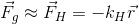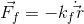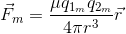## Magnetic Pendulum Simulator

### Parameters

Mass of pendulum: 0.01 kg

Magnet strength: 1000000 A/m

Friction: 0.1 kg/s

Gravity: 9.8 m/s2

Height of pendulum: 0.01 m

Initial magnets: 5

#### Instructions

• Red magnets are north poles. Blue magnets are south poles. Opposites attract!
• Doublie click on a magnet (or the pendulum) to change its polarity
• You can drag and drop both the magnets and the pendulum to your liking, even during the simulation.
• You can add a second pendulum (this is a great way to show the butterfly effect!).
• (If the app gets too slow, try clicking Reset Trace)

#### The Magnetic Pendulum

Physicists have always been fascinated by pendulums, and with good reason. They are very simple systems that can be easily understood, yet still have applications in almost all fields of physics. While they might be nothing more than a mass at the end of a string, modeling their movement has been used to solve many problems, from keeping time inside clocks to understanding quantum mechanics!
This all changes, however, when a magnet is applied at the end of such pendulums. Indeed, this transforms an object that is otherwise completely understood into a concrete example of the butterfly effect. This effect can be summarized as "small causes can have large consequences". This idea ties into a much broader and complex concept of mathematics: chaos theory.
This simulation features the physics behind a magnetic pendulum. Notice how modifying any setting or initial magnet location (even slightly) might result in changing the magnet over which the pendulum might end in an unpredictable manner. This is the butterfly effect! Try adding other magnets and changing the polarities to simulate the interesting motions of the pendulum. You can also include another pendulum to show how a slight change in the initial coordinates of a pendulum can have a big impact on its trajectory.

#### Simplifications

In order to simulate this experiment, the following simplifications were made:

• The pendulum is treated as a mass attached to a spring moving in a 2D plane. This is the small angle approximation: it mimicks closely the behavior of a pendulum with a very long string
• The magnets are treated as magnetic monopoles. While such monopoles have never been observed experimentally in nature, they provide a great model to simulate magnetic forces. Indeed, they remove the need to know the magnets' geometry to compute the magnetic force.

#### Physical Formulas

This entire simulation is based on Newton's famous second law, which states that the acceleration of any part of a system is equal to the sum of the forces applied on it divided by its mass. There are three forces at play here: Gravity, Friction, and Magnetic attraction/repulsion. These are respectively expressed by the following equations:#### The team (from left to right)

• Félix Léger: 2nd year Masters student in Particle Physics at McGill University
• Amir Bawab: 3rd year Undergraduate in Computer Science at Concordia University
• Justin Léger: 2nd year Undergraduate in Software Engineering at Concordia University